Next: Fits to experimental data Up: Results Previous: Single-particle energies in function

## Regression coefficients

It is clear that results shown in Figs. 1-5 can only tentatively indicate the type of dependence of the s.p. energies on coupling constants. In order to have a better handle on this dependence in a multi-dimensional space of parameters, it is more efficient to directly consider the regression coefficients discussed in Sec. 2. For a better separation of central and SO effects, the regression analysis was performed independently for centroids and SO splittings of the s.p. levels,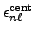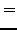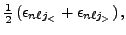(9)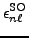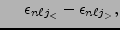(10)

respectively. Below we present selected results illustrating the main conclusions of the present study.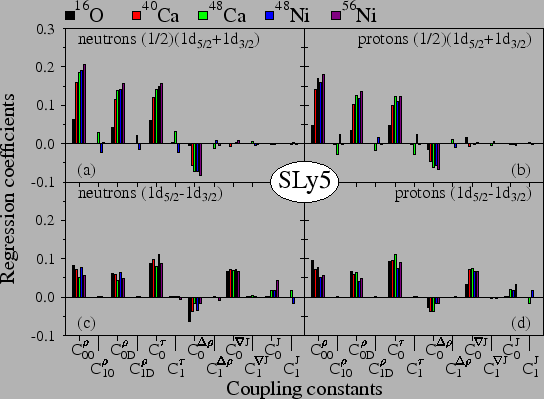Regression coefficients obtained for the centroids and SO splittings of the 1d levels in five light nuclei are shown in Fig. 6. As expected, in the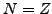nuclei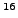O,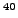Ca, and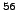Ni, the s.p. energies depend only on the isoscalar coupling constants. Only the centroids in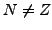nuclei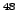Ca andNi weakly depend on the isovector coupling constants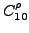,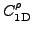, and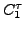, and the SO splittings in these nuclei weakly depend on the isovector tensor coupling constant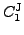. In all cases, dependence on the isovector SO coupling constant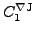is extremely weak. This illustrates the fact that the isospin excess inCa andNi is still not large enough for a pronounced dependence on the isovector coupling constants, and thus these coupling constants can be reasonably fixed only by going to even more exotic nuclei.

Centroids of the 1d levels depend predominantly on four isoscalar coupling constants,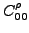,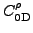,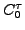, and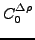. These coupling constants act on positions of centroids in a fairly similar way, i.e., by looking at positions of 1d orbitals in different nuclei one is not able to distinguish between central, effective mass, and surface effects, or differentiate between the density-dependent and density-independent coupling constants.

In all studied nuclei we obtain a standard uniform dependence of the SO splittings on the isoscalar SO coupling constant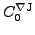. A somewhat weaker, but non-negligible, dependence on the isoscalar tensor coupling constant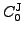is also clearly seen in spin-non-saturated nucleiCa,Ni, andNi. An unexpected result of our study is the fact that the SO splittings also very strongly depend on the central,and, effective-mass,, and surface,coupling constants. These dependencies were not explicitly recognized in the literature, because most often the SO splittings were adjusted at the end of the fitting protocol, i.e., for fixed values of other coupling constants. It is clear, however, that a global adjustment will create specific inter-relations between the SO and tensor coupling constants on one side and all the other ones on the other side. Relations of these kind between the SO and effective-mass effects were recently analyzed in Ref. .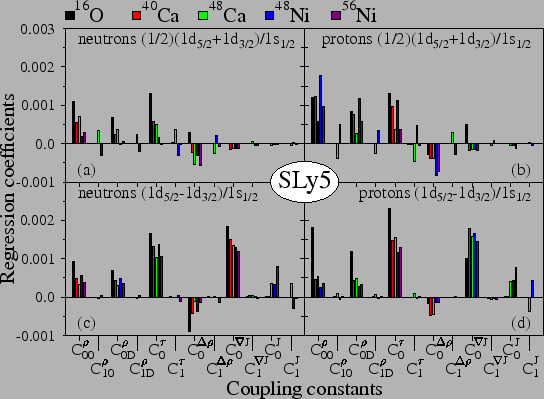In order to elucidate origins of the obtained dependencies of the SO splittings on the coupling constants, in Fig. 7 we show results of the regression analysis performed for the s.p. energies relative to those of the 1s orbital, i.e., in analogy with Eq. (8),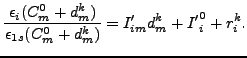(11)

In this way, we remove the overall effects of scaling of the s.p. spectra related to changing the depth of the central potential and/or effective mass.

One can see that, indeed, in this changed representation, dependence of relative centroids on coupling constants becomes significantly weaker. Also dependence of the relative SO splittings on the central,and, and surface,, coupling constants becomes less pronounced. Nevertheless, the relative SO splittings still quite strongly depend on the effective-mass coupling constant,, i.e., this dependence cannot be attributed to a simple scaling of the s.p. spectrum.

This fact is already clearly visible in Fig. 3, where the s.p. energies of favored SO partners, 1d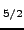and 1f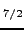, clearly follow that of the 2s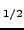level, while those of the unfavored SO partners, 1d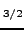and 1f, behave quite differently.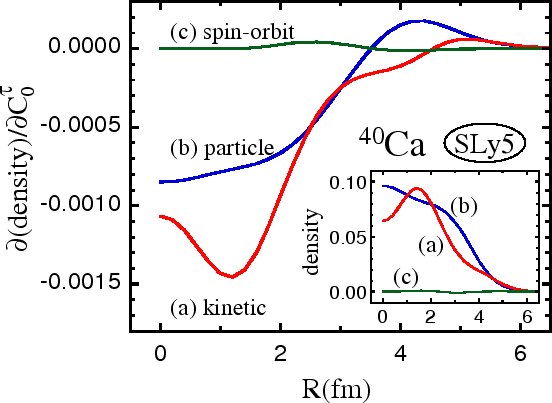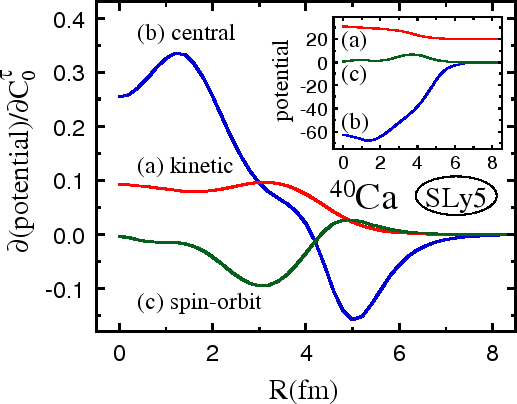The influence of the isoscalar effective-mass coupling constant on the SO splittings can be understood by inspecting the self-consistent densities and potentials. In Fig. 8 we show derivatives of the kinetic (a), particle (b), and SO (c) neutron densities inCa with respect to the coupling constant. For completeness, the inset shows the total densities. The derivatives were determined from differences of densities calculated self-consistently at two values close to the SLy5 value of. One can see that an increase innot only lowers the kinetic density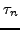, but also significantly lowers the particle density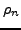in the center of the nucleus and moves the particles to the surface. As a result, it induces a smaller gradient of the particle density at the surface, and hence a weaker SO potential, as shown in Fig. 9, and as a consequence - a smaller SO splitting.

One can say that the inter-relations between the effective-mass and SO effects stem from the fact that EDF of Eq. (3) contains the effective-mass term,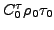, that depends on the product of the particle and kinetic densities. Therefore, changes in the effective-mass coupling constant induce changes in both these densities, and, in particular, they induce changes in the gradient of the particle density that affect the SO splittings. The role of the effective-mass term is clearly not restricted to changing the overall density of levels, as is the case in a trivial case of the infinite square-well potential, cf. also discussion in Ref. .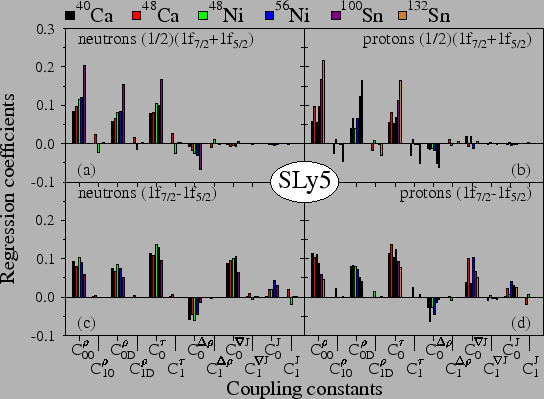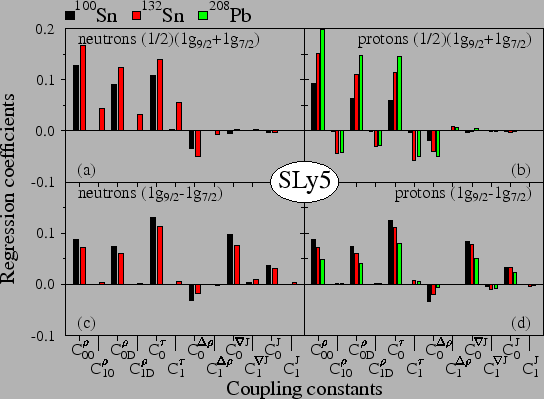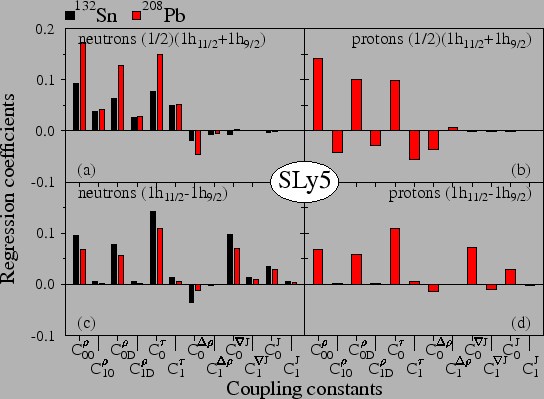Conclusions drawn from the results obtained for the 1d levels are further corroborated by those for the 1f, 1g, and 1h levels, which are shown in Figs. 10, 11, and 12, respectively. One can see that a tangible influence of the isovector coupling constants on centroids is obtained only in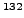Sn and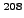Pb, i.e., in systems with a significant neutron excess. But even there the influence of the isovector coupling constants on the SO splittings is quite weak. Again we see that adjustment of the isovector coupling constants to empirical data may require studying systems much further away from stability.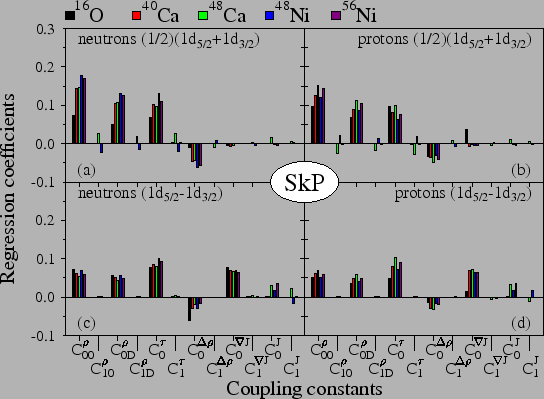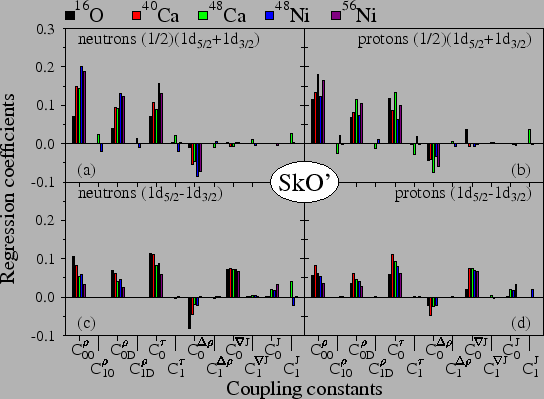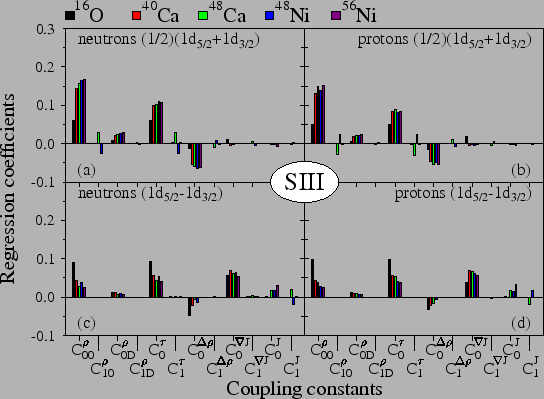A universality of the regression coefficients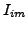, Eq. (8), is illustrated in Figs. 13, 14, and 15, in which we present results obtained for the SkP, SkO', and SIII Skyrme functionals, respectively. Comparison with the SLy5 results previously presented in Fig. 6, shows that for all the four functionals, patterns of regressions coefficients are very similar indeed. Small differences in the overall magnitudes are related to differences in the powers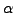of the density dependencies, Eq. (4), which are equal to 1/6, 1/4, and 1 for SLy5 and SkP, SkO', and SIII functionals, respectively. This is particularly clear for the coupling constants of density-dependent terms,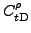, where the power of saturation density enters as a simple scale factor. For these functionals, values of the nuclear-matter effective masses are also different, and they read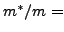0.7, 1, 0.9, and 0.76, respectively.Next: Fits to experimental data Up: Results Previous: Single-particle energies in function
Jacek Dobaczewski 2008-05-18